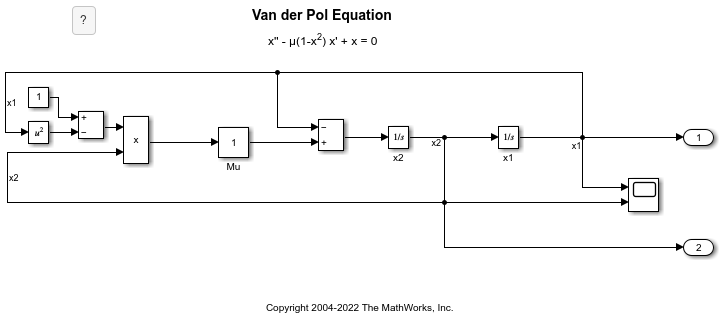Create and Edit Annotations Programmatically

Annotations are visual elements that you can use to add descriptive notes and callouts to your model. In addition to text-only annotations, you can create annotations that:

• Open websites

• Perform MATLAB® commands

• Display images

• Visually differentiate areas of block diagrams

The following examples show how to programmatically create, edit, and delete annotations.

Create Annotation Programmatically

Programmatically create, modify, and view an annotation.

Open a new model.

open_system(new_system)

Create an annotation with default properties using the Simulink.Annotation function.

a = Simulink.Annotation(gcs,'This is an annotation.');

After creating the annotation, use dot notation to set property values. For example, apply an 18-point font and light blue background to the annotation.

a.FontSize = 18;
a.BackgroundColor = 'lightBlue';

To view and briefly highlight the new annotation, use the view function.

view(a)

Programmatically Find and Modify Existing Annotations

Programmatically find and modify the properties of an annotation.

Open the vdp model.

vdp

To find the annotations in the model, use the find_system function.

h = find_system(gcs,'FindAll','on','Type','annotation');

To identify the annotations, query the text inside the annotations by using the get_param function.

get_param(h,'PlainText')
ans = 2x1 cell
{'van der Pol Equation'                   }

Suppose you want to apply a light blue background color to the 'van der Pol Equation' annotation.

Get the Simulink.Annotation object by specifying the corresponding index of the array.

a = get_param(h(2),'Object');

Use dot notation to set the value of the BackgroundColor property.

a.BackgroundColor = 'lightBlue';

Delete Annotation

Programmatically delete an annotation.

Open the vdp model.

vdp

To get the handles for the annotations in the model, use the find_system function.

h = find_system(gcs,'FindAll','on','Type','annotation');

To identify the annotations, query the text inside the annotations.

get_param(h,'PlainText')
ans = 2x1 cell
{'van der Pol Equation'                   }

To delete the title of the model ('van der Pol Equation'), get the Simulink.Annotation object that corresponds to the second handle.

a = get_param(h(2),'Object');

Delete the annotation from the model.

delete(a)

For rich-text annotations, you can use HTML formatting to add a hyperlink to text within the annotation.

Open a new model.

open_system(new_system)

Create two annotations, moving one of the annotations so that it does not overlap the other.

a1 = Simulink.Annotation(gcs,'This is an annotation.');
a2 = Simulink.Annotation(gcs,'This is another annotation.');
a2.Position = [0 20 28 34];

To create a hyperlink in the annotation, set Interpreter to 'rich' and define the hyperlink in the Text property.

a1.Interpreter = 'rich';
a1.Text = 'Go to <a href="www.mathworks.com">www.mathworks.com</a>.';

You can also embed MATLAB functions in the hyperlink.

a2.Interpreter = 'rich';
a2.Text = '<a href="matlab:magic(4)">Generate magic square</a>.';

Add an image to your model, such as a logo, by creating an image-only annotation.

Open a new model and create an annotation in it.

open_system(new_system)
a = Simulink.Annotation(gcs,'This is an annotation.');

Change the annotation to display only the specified image.

img = fullfile(matlabroot,'toolbox','matlab','imagesci','peppers.png');
setImage(a,img)

Create Area Programmatically

Create an area annotation in a model.

Open the vdp model.

open_system('vdp')

Create an area that includes some of the blocks in the model.Create and Hide Markup Annotation

To create annotations that can be easily hidden, create markup annotations.

Open a new model.

open_system(new_system)

Create two annotations, and move the second annotation so that it does not overlap the first annotation.

a1 = Simulink.Annotation(gcs,'This is a model annotation.');
a2 = Simulink.Annotation(gcs,'This is a markup annotation.');
a2.Position = [0 20 28 34];

By default, you create model annotations, which appear in the model.

Change the second annotation to a markup annotation.

a2.MarkupType = 'markup';

Configure the current model to hide markup annotations.

set_param(gcs,'ShowMarkup','off');

Both annotations remain, despite the markup annotation being hidden.

ah = find_system(gcs,'FindAll','on','Type','annotation');
at = get_param(ah,'Text')
at = 2x1 cell
{'This is a markup annotation.'}
{'This is a model annotation.' }

Find Annotation Executing Callback Function

If an annotation invoked a currently executing callback function, use the getCallbackAnnotation to determine which annotation invoked it. The function returns the corresponding Annotation object. This function is also useful if you write a callback function in a separate MATLAB file that contains multiple callback calls.